## Research and Reports on Mathematics

All submissions of the EM system will be redirected to Online Manuscript Submission System. Authors are requested to submit articles directly to Online Manuscript Submission System of respective journal.

Research Article, Res Rep Math Vol: 1 Issue: 1

# A Simpler Proof of the Characterization of Quadric CMC Hypersurfaces in Sn+1

Aquino CP1* and De Lima HF2

1Department of Mathematics, Universidade Federal do Piaui, Teresina, Brazil

2Department of Mathematics, Universidade Federal de Campina Grande, Campina Grande, Paraiba, Brazil

*Corresponding Author : Aquino CP
Department of Mathematics, Universidade Federal do Piaui, Teresina, Brazil
Tel: (86) 3215-5525
E-mail: cicero.aquino@ufpi.edu.br

Received: August 10, 2017 Accepted: September 01, 2017 Published: September 07, 2017

Citation: Aquino CP, De Lima HF (2017) A Simpler Proof of the Characterization of Quadric CMC Hypersurfaces in Sn+1. Res Rep Math 1:1.

## Abstract

In this short article, we present a new and simpler proof of a characterization of the quadric constant mean curvature hypersurfaces of the Euclidean sphere Sn+1, originally due to Alias, Brasil and Perdomo.

## Introduction

In 2008, Alias, Brasil and Perdomo studied complete hypersurfaces immersed in the unit Euclidean sphere, whose height and angle functions with respect to a fixed nonzero vector of the Euclidean space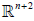are linearly related. Let us recall that, for a fixed arbitrary vectorthe height and the angle functions naturally attached to a hypersurfaceendowed with an orientation ν are defined, respectively, byandIn this setting, they showed the following characterization result concerning the quadric constant mean curvature hypersurfaces of Sn+1 [1,2]:

Theorem 1

Let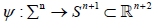be a complete hypersurface immersed in Sn+1 with constant mean curvature. la=λ fa for some non-zero vectorand some real number λ, then Σn is either a totally umbilical hypersurface or a Clifford torus, for some k = 0; 1;..; n and some k=0,1,…,n and ρ>0.

Later on, working with a different approach of that used in , the first and second authors characterized the totally umbilical and the hyperbolic cylinders of the hyperbolic space Hn+1as the only complete hypersurfaces with constant mean curvature and whose support functions with respect to a fixed nonzero vector a of the Lorentz- Minkowski space are linearly related (see Theorem 4:1 of [3,4], for the case that a is either space like or time like, and Theorem 4:2 of , for the case that a is a nonzero null vector). In this short article, our purpose is just to use a similar approach of that in [4,5] in order to present a new and more simple proof of Theorem 1 (cf. Section 3). For this, in Section 2 we recall some preliminaries facts concerning hypersurfaces immersed in Sn+1.

Preliminaries

Let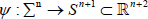be an orientable hypersurface immersed in the Euclidean sphere. We will denote by A the Weingarten operator of Σn with respect to a globally defined unit normal vector ν.

In order to set up the notation, let us represent by ∇0 , ∇and ∇ the Levi-Civita connections of, Sn+1 and Σn respectively. Then the Gauss and Weingarten formulas for Σn in Sn+1 are given, respectively, byandfor all tangent vector fieldsIn what follows, we will work with the first three symmetric elementary functions of the principal curvatures λ1,… λn of ψ, namely: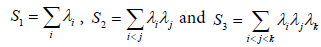where i, j, k ∈ {1,…, n}.

As before, for a fixed arbitrary vector alet us consider the height and the angle functions naturally attached to which are defined, respectively, byand. A direct computation allows us to conclude that the gradient of such functions are given byand, where aΤ is the orthogonal projection of a onto the tangent bundle Τ Σ , that is,Taking into account thatand using Gauss and Weingarten formulas, we obtainfor all. We use this previous identity jointly with Codazzi equation to deduce thatFor all thatThus according to  (see also ), it follows from the last two identities that(2.1)(2.2)

where H = (1/n) S1 is the mean curvature function of Σn

For what follows, it is convenient to consider the so-called Newton transformation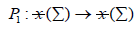P1= S1-A

where I is the identity operator. Naturally associated with the Newton transformation P1, we have the Cheng-Yau’s square operator , which is the second order linear di erential operatorgiven by(2.4)

Herestands for the self-adjoint linear operator metrically equivalent to the hessian of h, and it is given byFor all X, Y∈ x (Σ) .

Based on Reilly’s seminal paper [8-10], Rosenberg  showed the following idenfitities related to the action of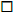on the functions la and fa:(2.5)

And(2.6)

To close this section, we quote a suitable Simons-type formula which can be found in  or .(2.7)

Proof of Theorem

Now, we are in position to proceed with our alternative proof of Theorem 1.1. If λ=0 then la= λfa=0, that isfor alland, consequently, Σn is a totally umbilical sphere of Sn+1.

So, let us assume that λ≠0. We have Δla=λΔfa and using the fact that H is constant, from (2.1) and (2.2) we conclude that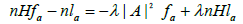Or equivalently,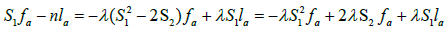Hence, we get that(3.1)

By (3.1), we obtainThus,(3.2)

We define a functionby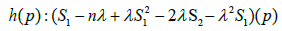Suppose that h(p0)≠0 for some p0 ∈ Σn Since h is smooth, there exists a neighbourhood u of p0 in Σn in which h(p)≠0 all p∈ u From (3.2) we conclude that la=0 in u and, hence fa=0 in u. since λ≠0. we arrive at a contradiction because in Σn we haveTherefore, h = 0 on Σn, that is,(3.3)

Consequently, S2 is constant on Σn . Repeating the previous argument for the operator L1 and using the fact that S2 is constant, we also obtain that(3.4)

We observe that the above equation shows that S3 is also constant on Σn . We also note that this argument shows, in fact, that Sr is a constant function on Σn for all 2≤r≤n. From (2.7) we getMore precisely,(3.5)

We observe that if H=0, then S1=0 and, consequently, |A|2=−2s2 From (3.3), we have 2S2 =−n and |A|2 = n. Therefore, sinceWe have thatand, hence, from Theorem 4 of , we conclude that Σn must be a Clifford torus, for some k=0,1,…,n and some ρ>0.

Now, suppose that H≠0 By equation (3.4) we getthat is,(3.7)

From equation (3.5) we have(3.8)

Furthermore, from a straightforward computation we can verify that(3.9)

Hence, if S2 = 0 we obtain of (3.9) thatconsequently, |∇A|2 =0 and, since Σn is complete, it follows once more from Theorem 4 of  that Σn must be a Clifford torus.

If S2≠0 then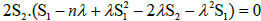implies(3.10)

We note that (3.10) and (3.9) implyand, hence, repeating the previous argument we also get that Σn is a Clifford torus. Therefore, we conclude the proof of Theorem 1.

## References